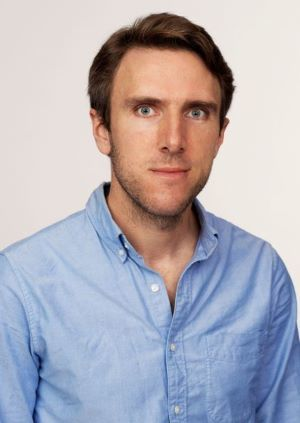# Ben Ward

###Assistant Professor

Phone: 419-372-7472
Email: benward@bgsu.edu
Address: Office: 427 Mathematical Sciences Building
Department of Mathematics and Statistics
Bowling Green State University
Bowling Green, OH 43403-0206

### Education

Ph.D. Purdue University

### Publications

All papers are available on the arXiv. See: https://arxiv.org/search/?searchtype=author&query=Ward%2C+B+C

• Koszul Feynman Categories. Submitted 2021 [with R. Kaufmann].

Summary: Proves that every cubical Feynman category is Koszul.

• Wheel Graph Homology Classes via Lie Graph Homology. Submitted 2021.

Summary: Constructs representatives of the wheel graph homology classes in a Lie graph homology model of the Grothendieck-Teichmuller Lie algebra.

• Toward a minimal model for $H_\ast(\overline{\mathcal{M}})$. Submitted 2021.

Summary: Constructs the first family of Massey products on the twisted modular operad of the homologies of moduli spaces of punctured surfaces, and interprets them in terms of the minimal model for the homology of their compactifications.

• Massey Products for graph homology. To appear in Int. Math. Res. Not. IMRN 2021.

Summary: Develops a notion of Massey products for modular operads and uses the analogs of spectral sequences in rational homotopy theory to do several calculations in graph homology. The main technical result shows that the operad encoding modular operads is Koszul.

• Intertwining for semi-direct product operads. Algebr. Geom. Topol., 19(4):1903--1934, 2019.

Summary: Proves a Koszul duality result relating different ways to use the $SO(n)$ action on configurations of points in $\mathbb{R}^n$ to produce new operads. Also considers implications for moduli spaces of punctured spheres in the case $n=2$.}

• Six operations formalism for generalized operads. Theory Appl. Categ. vol. 34 no. 6, 121-169, 2019.

Summary: Shows that Koszul duality for algebras and operads is governed by the same categorical structure as Verdier duality in sheaf theory.

• Gravity formality. Adv. Math., (331):439-483, 2018. [with R. Campos]

Summary: Extends Kontsevich's cyclic formality theorem from an equivalence of Lie algebras to an equivalence of algebras over the moduli spaces of punctured spheres.

• Feynman categories. Asterisque, (387):vii+161, 2017. [with R. Kaufmann]

Summary: Gives a new categorical foundation for operads and their algebras. Applications to homotopy invariant algebraic structures in the language of model categories.

• Hypercommutative algebras and cyclic cohomology. Michigan Math. J., 66(3):533--547, 2017.

Summary: Constructs a chain model for the operad of Deligne-Mumford compactifications of punctured genus $0$ curves. Shows this chain model acts on the Hochschild complex in the presence of certain degeneration data.

• Maurer-{C}artan elements and cyclic operads. J. Noncommut. Geom., 10(4):1403--1464, 2016.

Summary: Constructs a chain model for the operad of moduli spaces of punctured spheres. Shows this chain model acts on the cyclic cochains of certain $A_\infty$ algebras.

• The odd origin of {G}erstenhaber brackets, BV operators, and master equations. J. Math. Phys., 56(10):103504, 40pp, 2015. [with R. Kaufmann and J. Zuniga]

Summary: Gives a unifying construction of Lie brackets associated to certain classes of graphs. Explores the role of compatible cup products and gives an interpretation of Maurer-Cartan elements.

• Cyclic $A_\infty$ structures and Deligne's conjecture. Algebr. Geom. Topol., 12(3):1487--1551, 2012.

Summary: Generalizes known proofs of Deligne's conjecture due to Kontsevich-Soibelman and Kaufmann to the context of cyclic $A_\infty$ algebras and categories.

Updated: 01/12/2022 11:04PM